# colorspace/colorspace.h File Reference

This graph shows which files directly or indirectly include this file: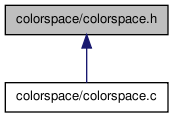Go to the source code of this file.

## Classes

struct  colortransform
struct for representing a color transform More...

## Defines

#define WHITEPOINT_X   0.950456
XYZ color of the D65 white point.
#define WHITEPOINT_Y   1.0
#define WHITEPOINT_Z   1.088754

## Typedefs

typedef double num
Datatype to use for representing real numbers Set this typedef to either double or float depending on the application.

## Functions

int GetColorTransform (colortransform *Trans, const char *TransformString)
Given a transform string, returns a colortransform struct.
void ApplyColorTransform (colortransform Trans, num *D0, num *D1, num *D2, num S0, num S1, num S2)
Apply a colortransform.
void Rgb2Yuv (num *Y, num *U, num *V, num R, num G, num B)
Convert sRGB to NTSC/PAL Y'UV Luma + Chroma.
void Yuv2Rgb (num *R, num *G, num *B, num Y, num U, num V)
Convert NTSC/PAL Y'UV to sRGB.
void Rgb2Ycbcr (num *Y, num *Cb, num *Cr, num R, num G, num B)
sRGB to Y'CbCr Luma + Chroma
void Ycbcr2Rgb (num *R, num *G, num *B, num Y, num Cb, num Cr)
Y'CbCr to sRGB.
void Rgb2Jpegycbcr (num *R, num *G, num *B, num Y, num Cb, num Cr)
sRGB to JPEG-Y'CbCr Luma + Chroma
void Jpegycbcr2Rgb (num *R, num *G, num *B, num Y, num Cb, num Cr)
JPEG-Y'CbCr to sRGB.
void Rgb2Ypbpr (num *Y, num *Pb, num *Pr, num R, num G, num B)
sRGB to Y'PbPr Luma (ITU-R BT.601) + Chroma
void Ypbpr2Rgb (num *R, num *G, num *B, num Y, num Pb, num Pr)
Y'PbPr to sRGB.
void Rgb2Ydbdr (num *Y, num *Db, num *Dr, num R, num G, num B)
sRGB to SECAM Y'DbDr Luma + Chroma
void Ydbdr2Rgb (num *R, num *G, num *B, num Y, num Db, num Dr)
SECAM Y'DbDr to sRGB.
void Rgb2Yiq (num *Y, num *I, num *Q, num R, num G, num B)
sRGB to NTSC YIQ
void Yiq2Rgb (num *R, num *G, num *B, num Y, num I, num Q)
Convert NTSC YIQ to sRGB.
void Rgb2Hsv (num *H, num *S, num *V, num R, num G, num B)
Convert an sRGB color to Hue-Saturation-Value (HSV).
void Hsv2Rgb (num *R, num *G, num *B, num H, num S, num V)
Convert a Hue-Saturation-Value (HSV) color to sRGB.
void Rgb2Hsl (num *H, num *S, num *L, num R, num G, num B)
Convert an sRGB color to Hue-Saturation-Lightness (HSL).
void Hsl2Rgb (num *R, num *G, num *B, num H, num S, num L)
Convert a Hue-Saturation-Lightness (HSL) color to sRGB.
void Rgb2Hsi (num *H, num *S, num *I, num R, num G, num B)
Convert an sRGB color to Hue-Saturation-Intensity (HSI).
void Hsi2Rgb (num *R, num *G, num *B, num H, num S, num I)
Convert a Hue-Saturation-Intesity (HSI) color to sRGB.
void Rgb2Xyz (num *X, num *Y, num *Z, num R, num G, num B)
Transform sRGB to CIE XYZ with the D65 white point.
void Xyz2Rgb (num *R, num *G, num *B, num X, num Y, num Z)
Transform CIE XYZ to sRGB with the D65 white point.
void Xyz2Lab (num *L, num *a, num *b, num X, num Y, num Z)
void Lab2Xyz (num *X, num *Y, num *Z, num L, num a, num b)
void Xyz2Luv (num *L, num *u, num *v, num X, num Y, num Z)
void Luv2Xyz (num *X, num *Y, num *Z, num L, num u, num v)
void Xyz2Lch (num *L, num *C, num *H, num X, num Y, num Z)
void Lch2Xyz (num *X, num *Y, num *Z, num L, num C, num H)
void Xyz2Cat02lms (num *L, num *M, num *S, num X, num Y, num Z)
XYZ to CAT02 LMS.
void Cat02lms2Xyz (num *X, num *Y, num *Z, num L, num M, num S)
CAT02 LMS to XYZ.
void Rgb2Lab (num *L, num *a, num *b, num R, num G, num B)
void Lab2Rgb (num *R, num *G, num *B, num L, num a, num b)
void Rgb2Luv (num *L, num *u, num *v, num R, num G, num B)
void Luv2Rgb (num *R, num *G, num *B, num L, num u, num v)
void Rgb2Lch (num *L, num *C, num *H, num R, num G, num B)
void Lch2Rgb (num *R, num *G, num *B, num L, num C, num H)
void Rgb2Cat02lms (num *L, num *M, num *S, num R, num G, num B)
void Cat02lms2Rgb (num *R, num *G, num *B, num L, num M, num S)

## Detailed Description

Definition in file colorspace.h.

## Define Documentation

 #define WHITEPOINT_X   0.950456

XYZ color of the D65 white point.

Definition at line 20 of file colorspace.h.

 #define WHITEPOINT_Y   1.0

Definition at line 21 of file colorspace.h.

 #define WHITEPOINT_Z   1.088754

Definition at line 22 of file colorspace.h.

## Typedef Documentation

 typedef double num

Datatype to use for representing real numbers Set this typedef to either double or float depending on the application.

Definition at line 16 of file colorspace.h.

## Function Documentation

 void ApplyColorTransform ( colortransform Trans, num * D0, num * D1, num * D2, num S0, num S1, num S2 )

Apply a colortransform.

Parameters:
 Trans colortransform struct created by GetColorTransform D0,D1,D2 pointers to hold the result S0,S1,S2 the input values

Definition at line 1191 of file colorspace.c.

 void Cat02lms2Rgb ( num * R, num * G, num * B, num L, num M, num S )

Definition at line 964 of file colorspace.c.

Here is the call graph for this function: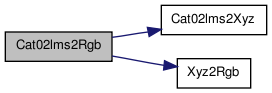void Cat02lms2Xyz ( num * X, num * Y, num * Z, num L, num M, num S )

CAT02 LMS to XYZ.

Definition at line 897 of file colorspace.c.

Here is the caller graph for this function:int GetColorTransform ( colortransform * Trans, const char * TransformString )

Given a transform string, returns a colortransform struct.

Parameters:
 Trans a colortransform pointer to hold the transform TransformString string specifying the transformations
Returns:
1 on success, 0 on failure

This function provides a convenient interface to the collection of transform functions in this file. TransformString specifies the source and destination color spaces, TransformString = "dest<-src" or alternatively, TransformString = "src->dest".

Supported color spaces are "RGB" sRGB Red Green Blue (ITU-R BT.709 gamma-corrected), "YPbPr" Luma (ITU-R BT.601) + Chroma, "YCbCr" Luma + Chroma ("digitized" version of Y'PbPr), "JPEG-YCbCr" Luma + Chroma space used in JFIF JPEG, "YUV" NTSC PAL Y'UV Luma + Chroma, "YIQ" NTSC Y'IQ Luma + Chroma, "YDbDr" SECAM Y'DbDr Luma + Chroma, "HSV" or "HSB" Hue Saturation Value/Brightness, "HSL" or "HLS" Hue Saturation Luminance, "HSI" Hue Saturation Intensity, "XYZ" CIE XYZ, "Lab" CIE L*a*b* (CIELAB), "Luv" CIE L*u*v* (CIELUV), "LCH" CIE L*C*H* (CIELCH), "CAT02 LMS" CIE CAT02 LMS. Color space names are case-insensitive and spaces are ignored. When sRGB is the source or destination, it can be omitted. For example "yuv<-" is short for "yuv<-rgb".

The routine returns a colortransform structure representing the transform. The transform is performed by calling GetColorTransform. For example,

```       num S = {173, 0.8, 0.5};
num D;
colortransform Trans;

if(!(GetColorTransform(&Trans, "HSI -> Lab")))
{
printf("Invalid syntax or unknown color space\n");
return;
}

ApplyColorTransform(Trans, &D, &D, &D, S, S, S);
```

Definition at line 1067 of file colorspace.c.

Here is the call graph for this function: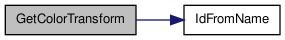void Hsi2Rgb ( num * R, num * G, num * B, num H, num S, num I )

Convert a Hue-Saturation-Intesity (HSI) color to sRGB.

Parameters:
 R,G,B pointers to hold the result H,S,I the input HSI values

The input values are assumed to be scaled as 0 <= H < 360, 0 <= S <= 1, 0 <= I <= 1. The output sRGB values are scaled between 0 and 1. This is the inverse transformation of Rgb2Hsi.

Wikipedia: http://en.wikipedia.org/wiki/HSL_and_HSV

Definition at line 658 of file colorspace.c.

 void Hsl2Rgb ( num * R, num * G, num * B, num H, num S, num L )

Convert a Hue-Saturation-Lightness (HSL) color to sRGB.

Parameters:
 R,G,B pointers to hold the result H,S,L the input HSL values

The input values are assumed to be scaled as 0 <= H < 360, 0 <= S <= 1, 0 <= L <= 1. The output sRGB values are scaled between 0 and 1. This is the inverse transformation of Rgb2Hsl.

Wikipedia: http://en.wikipedia.org/wiki/HSL_and_HSV

Definition at line 558 of file colorspace.c.

Here is the caller graph for this function: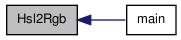void Hsv2Rgb ( num * R, num * G, num * B, num H, num S, num V )

Convert a Hue-Saturation-Value (HSV) color to sRGB.

Parameters:
 R,G,B pointers to hold the result H,S,V the input HSV values

The input values are assumed to be scaled as 0 <= H < 360, 0 <= S <= 1, 0 <= V <= 1. The output sRGB values are scaled between 0 and 1. This is the inverse transformation of Rgb2Hsv.

Wikipedia: http://en.wikipedia.org/wiki/HSL_and_HSV

Definition at line 446 of file colorspace.c.

 void Jpegycbcr2Rgb ( num * R, num * G, num * B, num Y, num Cb, num Cr )

JPEG-Y'CbCr to sRGB.

Definition at line 313 of file colorspace.c.

Here is the call graph for this function: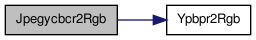void Lab2Rgb ( num * R, num * G, num * B, num L, num a, num b )

Definition at line 917 of file colorspace.c.

Here is the call graph for this function: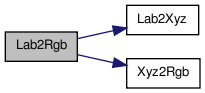void Lab2Xyz ( num * X, num * Y, num * Z, num L, num a, num b )

Convert CIE L*a*b* (CIELAB) to CIE XYZ with the D65 white point

Parameters:
 X,Y,Z pointers to hold the result L,a,b the input L*a*b* values

Definition at line 782 of file colorspace.c.

Here is the caller graph for this function: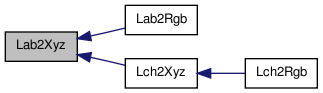void Lch2Rgb ( num * R, num * G, num * B, num L, num C, num H )

Definition at line 948 of file colorspace.c.

Here is the call graph for this function: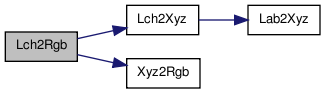void Lch2Xyz ( num * X, num * Y, num * Z, num L, num C, num H )

Convert CIE L*C*H* to CIE XYZ with the D65 white point

Parameters:
 X,Y,Z pointers to hold the result L,C,H the input L*C*H* values

Definition at line 877 of file colorspace.c.

Here is the call graph for this function: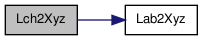Here is the caller graph for this function: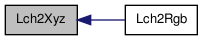void Luv2Rgb ( num * R, num * G, num * B, num L, num u, num v )

Definition at line 933 of file colorspace.c.

Here is the call graph for this function: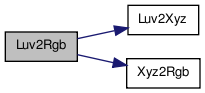void Luv2Xyz ( num * X, num * Y, num * Z, num L, num u, num v )

Convert CIE L*u*v* (CIELUV) to CIE XYZ with the D65 white point

Parameters:
 X,Y,Z pointers to hold the result L,u,v the input L*u*v* values

Definition at line 830 of file colorspace.c.

Here is the caller graph for this function: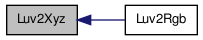void Rgb2Cat02lms ( num * L, num * M, num * S, num R, num G, num B )

Definition at line 956 of file colorspace.c.

Here is the call graph for this function: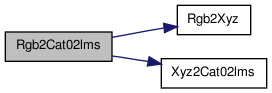void Rgb2Hsi ( num * H, num * S, num * I, num R, num G, num B )

Convert an sRGB color to Hue-Saturation-Intensity (HSI).

Parameters:
 H,S,I pointers to hold the result R,G,B the input sRGB values scaled in [0,1]

This routine transforms from sRGB to the cylindrical HSI color space. The sRGB values are assumed to be between 0 and 1. The output values are H = polar hue angle (0 <= H < 360), S = 1 - min(R',G',B')/I (0 <= S <= 1), I = (R'+G'+B')/3 (0 <= I <= 1). The inverse color transformation is given by Hsi2Rgb.

Wikipedia: http://en.wikipedia.org/wiki/HSL_and_HSV

Definition at line 622 of file colorspace.c.

 void Rgb2Hsl ( num * H, num * S, num * L, num R, num G, num B )

Convert an sRGB color to Hue-Saturation-Lightness (HSL).

Parameters:
 H,S,L pointers to hold the result R,G,B the input sRGB values scaled in [0,1]

This routine transforms from sRGB to the double hexcone HSL color space The sRGB values are assumed to be between 0 and 1. The outputs are H = hexagonal hue angle (0 <= H < 360), S = { C/(2L) if L <= 1/2 (0 <= S <= 1), { C/(2 - 2L) if L > 1/2 L = (max(R',G',B') + min(R',G',B'))/2 (0 <= L <= 1), where C = max(R',G',B') - min(R',G',B'). The inverse color transformation is given by Hsl2Rgb.

Wikipedia: http://en.wikipedia.org/wiki/HSL_and_HSV

Definition at line 512 of file colorspace.c.

Here is the caller graph for this function: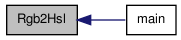void Rgb2Hsv ( num * H, num * S, num * V, num R, num G, num B )

Convert an sRGB color to Hue-Saturation-Value (HSV).

Parameters:
 H,S,V pointers to hold the result R,G,B the input sRGB values scaled in [0,1]

This routine transforms from sRGB to the hexcone HSV color space. The sRGB values are assumed to be between 0 and 1. The output values are H = hexagonal hue angle (0 <= H < 360), S = C/V (0 <= S <= 1), V = max(R',G',B') (0 <= V <= 1), where C = max(R',G',B') - min(R',G',B'). The inverse color transformation is given by Hsv2Rgb.

Wikipedia: http://en.wikipedia.org/wiki/HSL_and_HSV

Definition at line 400 of file colorspace.c.

 void Rgb2Jpegycbcr ( num * R, num * G, num * B, num Y, num Cb, num Cr )

sRGB to JPEG-Y'CbCr Luma + Chroma

Definition at line 305 of file colorspace.c.

Here is the call graph for this function: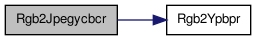void Rgb2Lab ( num * L, num * a, num * b, num R, num G, num B )

Definition at line 909 of file colorspace.c.

Here is the call graph for this function: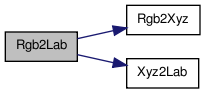void Rgb2Lch ( num * L, num * C, num * H, num R, num G, num B )

Definition at line 940 of file colorspace.c.

Here is the call graph for this function: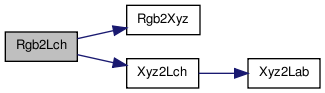void Rgb2Luv ( num * L, num * u, num * v, num R, num G, num B )

Definition at line 925 of file colorspace.c.

Here is the call graph for this function: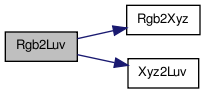void Rgb2Xyz ( num * X, num * Y, num * Z, num R, num G, num B )

Transform sRGB to CIE XYZ with the D65 white point.

Parameters:
 X,Y,Z pointers to hold the result R,G,B the input sRGB values

Poynton, "Frequently Asked Questions About Color," page 10 Wikipedia: http://en.wikipedia.org/wiki/SRGB Wikipedia: http://en.wikipedia.org/wiki/CIE_1931_color_space

Definition at line 704 of file colorspace.c.

Here is the caller graph for this function: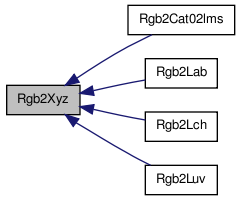void Rgb2Ycbcr ( num * Y, num * Cb, num * Cr, num R, num G, num B )

sRGB to Y'CbCr Luma + Chroma

Definition at line 284 of file colorspace.c.

 void Rgb2Ydbdr ( num * Y, num * Db, num * Dr, num R, num G, num B )

sRGB to SECAM Y'DbDr Luma + Chroma

Definition at line 340 of file colorspace.c.

 void Rgb2Yiq ( num * Y, num * I, num * Q, num R, num G, num B )

sRGB to NTSC YIQ

Definition at line 358 of file colorspace.c.

 void Rgb2Ypbpr ( num * Y, num * Pb, num * Pr, num R, num G, num B )

sRGB to Y'PbPr Luma (ITU-R BT.601) + Chroma

Definition at line 322 of file colorspace.c.

Here is the caller graph for this function: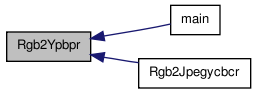void Rgb2Yuv ( num * Y, num * U, num * V, num R, num G, num B )

Convert sRGB to NTSC/PAL Y'UV Luma + Chroma.

Parameters:
 Y,U,V pointers to hold the result R,G,B the input sRGB values

Wikipedia: http://en.wikipedia.org/wiki/YUV

Definition at line 261 of file colorspace.c.

 void Xyz2Cat02lms ( num * L, num * M, num * S, num X, num Y, num Z )

XYZ to CAT02 LMS.

Definition at line 888 of file colorspace.c.

Here is the caller graph for this function: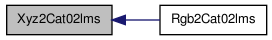void Xyz2Lab ( num * L, num * a, num * b, num X, num Y, num Z )

Convert CIE XYZ to CIE L*a*b* (CIELAB) with the D65 white point

Parameters:
 L,a,b pointers to hold the result X,Y,Z the input XYZ values

Definition at line 760 of file colorspace.c.

Here is the caller graph for this function: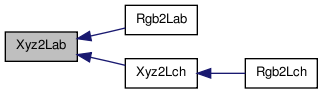void Xyz2Lch ( num * L, num * C, num * H, num X, num Y, num Z )

Convert CIE XYZ to CIE L*C*H* with the D65 white point

Parameters:
 L,C,H pointers to hold the result X,Y,Z the input XYZ values

CIE L*C*H* is related to CIE L*a*b* by a* = C* cos(H* pi/180), b* = C* sin(H* pi/180).

Definition at line 858 of file colorspace.c.

Here is the call graph for this function: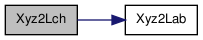Here is the caller graph for this function: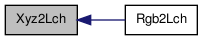void Xyz2Luv ( num * L, num * u, num * v, num X, num Y, num Z )

Convert CIE XYZ to CIE L*u*v* (CIELUV) with the D65 white point

Parameters:
 L,u,v pointers to hold the result X,Y,Z the input XYZ values

Definition at line 801 of file colorspace.c.

Here is the caller graph for this function: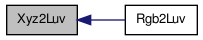void Xyz2Rgb ( num * R, num * G, num * B, num X, num Y, num Z )

Transform CIE XYZ to sRGB with the D65 white point.

Parameters:
 R,G,B pointers to hold the result X,Y,Z the input XYZ values

Official sRGB specification (IEC 61966-2-1:1999) Poynton, "Frequently Asked Questions About Color," page 10 Wikipedia: http://en.wikipedia.org/wiki/SRGB Wikipedia: http://en.wikipedia.org/wiki/CIE_1931_color_space

Definition at line 726 of file colorspace.c.

Here is the caller graph for this function: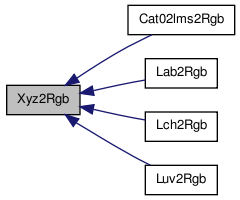void Ycbcr2Rgb ( num * R, num * G, num * B, num Y, num Cb, num Cr )

Y'CbCr to sRGB.

Definition at line 293 of file colorspace.c.

 void Ydbdr2Rgb ( num * R, num * G, num * B, num Y, num Db, num Dr )

SECAM Y'DbDr to sRGB.

Definition at line 349 of file colorspace.c.

 void Yiq2Rgb ( num * R, num * G, num * B, num Y, num I, num Q )

Convert NTSC YIQ to sRGB.

Definition at line 367 of file colorspace.c.

 void Ypbpr2Rgb ( num * R, num * G, num * B, num Y, num Pb, num Pr )

Y'PbPr to sRGB.

Definition at line 331 of file colorspace.c.

Here is the caller graph for this function: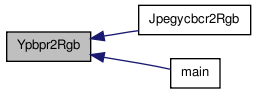void Yuv2Rgb ( num * R, num * G, num * B, num Y, num U, num V )

Convert NTSC/PAL Y'UV to sRGB.

Parameters:
 R,G,B pointers to hold the result Y,U,V the input YUV values

Definition at line 275 of file colorspace.c.

Generated on Wed Oct 5 14:39:21 2011 for local color correction by1.6.3